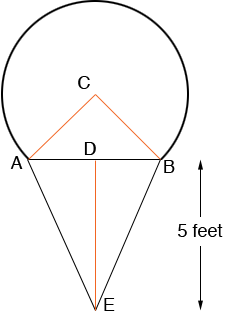SEARCH HOMEMath Central Quandaries & QueriesHi Please help me with the below question. The outline of a sign for an ice-cream store is made by placing 3/4 th of the circumference of a circle with radius 2 feet on top of an isosceles triangle with height 5 feet, as shown above. What is the perimeter, in feet, of the sign? (A) 3pi+ 3 sqrt3 (B) 3pi + 6 sqrt3 (C) 3pi + 2 sqrt 33 (D) 4pi + 3 sqrt3 (E) 4pi + 6 sqrt3 Thanks ArulHi Arul,The perimeter is the arc of the circle clockwise from A to B plus the line segments BE and EA. The length of the arc of the circle clockwise from A to B is three-quarters the circumference of the circle of radius 2. The line segment BE is congruent to the line segment EA so you need only find the length of one of them.

Since the angle BCA is one-quarter the way around a circle it is a right angle. Thus the triangle BCA is a right triangle and you can use Pythagoras' theorem to find the length of AB. Hence you can find the length of DB which is half the length of AB. Triangle BDE is also a right triangle and hence Pythagoras' theorem can be used again to find the length of BE.

PennyMath Central is supported by the University of Regina and The Pacific Institute for the Mathematical Sciences.